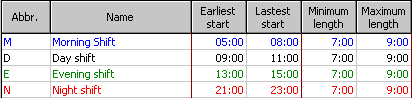Operating Hours Assistant A system for design of shifts Randomly generated problems We proposed three different sets of randomly generated examples for the shift design problem, which can be used further by other researchers to compare their results with ours. Additionally, Set 4 contains one real life example and two special examples modified from Set 3.    Set 1 of randomly generated problems  (download ) The basic idea for random examples is to generate for each shift type a specific number of time intervals which fulfill constraints about the start and length of the shifts. Each time interval corresponds to one legal shift. Randomly, 1-5 time intervals are generated for each shift type. The start of the time interval is generated randomly from the possible starts in the start region of a shift type and the length of time intervals is one random possible length (determined by the shift type). In Table 1 constraints for shift types are given. The time interval is taken randomly to be 15, 30 or 60 minutes.Table 1: Constraints about the shift types for the random generator Duties for each time interval are generated such that initially, for each time interval, a random number between 1-5 is generated with equal probability. The time interval will have assigned (as number of employees) this random number for each day during the week with probability 0.9 (probability that the duties for each particular day change from the standard value is 0.1). If this value should change for a particular day, the new value (1-5) is generated randomly for that day. During the weekend the probability that the value changes is 0.6. If the value should change, a new random number is generated (the probability that either Sunday or Saturday changes from this number is again 0.1 percent).  Weights for shortage and excess are 1, for number of shifts the weight is equal to the length of the time unit in minutes (15, 30, or 60) and for duties per week it is 1000. The maximum number of duties per week is set to 5. The average number of hours per week is 38,5. As we want to generate problems for which we can easily estimate the fitness in advance, if the duties per week exceed 5 (for optimal solution regarding excess, shortage and number of shifts), we put the weights of the duties per week to be 0. This means we consider indeed only minimizing the shortage, excess and number of shifts. Set 2 of randomly generated problems  (download ) Set 2 contains similar instances to Set 1, but here the `best known' solutions of instances 1 to 10 were constructed to feature 12 shifts, those of instances 11 to 20 to feature 16 shifts, and those of instances 21 to 30 to feature 20 shifts. This allows us to study the relation between the number of shifts in the `best known' solutions and the running times of the heuristics. While knowing these `best known' solutions eases the evaluation of the proposed heuristics, it also might form a biased preselection towards instances where zero deviation solutions exist for sure, thereby letting all or some of the heuristics behave in ways that are unusual for instances for which no such solution can be constructed. The remaining set is therefore composed of instances where with high likelihood solutions without deviations do not exist:  Set 3 of randomly generated problems  (download ) Set 3 contains instances without `best known' solutions. They were constructed with the same random instance generator as the two previous sets but allowing the constructed solutions to contain invalid shifts that deviate from normal starting times and lengths by up to 4 timeslots. The number of shifts is similar to those in Set 2, i.e., instances 1 to 10 feature 12 shifts (invalid and valid ones) etc. This construction ensures that it is unlikely that zero deviation solutions exist for these instances. It might also be of interest to see whether a significant difference in performance for some of the heuristics can be recognized compared to Set 2, which would provide evidence that the way Sets 1 and 2 were constructed constituted a bias for the heuristics.  Set 4 of problems  (download ) This set contains three instances: Instance one: This is a rather complicated real instance for comparison purposes to the randomly generated ones.  Instance two: This is instance number 5 from Set 3, this time with the length of its timeslot halved. This allows  us to study how the heuristics behave when the slotlength alone is varied. Instance three: This again is instance number 5 from Set  3,  an instance of intermediate difficulty, but with its workforce doubled. This allows us to study in which way the amount of workforce requirements influences the behavior of the heuristics.   Home mailto: {musliu,wsi}@dbai.tuwien.ac.at